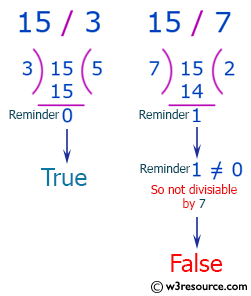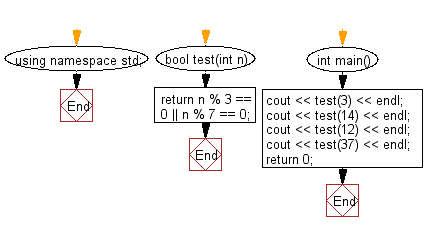﻿ C++ : Check if a given number is a multiple of 3 or 7# C++ Exercises: Check if a given positive number is a multiple of 3 or a multiple of 7

## C++ Basic Algorithm: Exercise-10 with Solution

Write a C++ program to check if a given positive number is a multiple of 3 or a multiple of 7.

Sample Solution:

C++ Code :

``````#include <iostream>
using namespace std;

bool test(int n)
{
return n % 3 == 0 || n % 7 == 0;
}

int main()
{
cout << test(3) << endl;
cout << test(14) << endl;
cout << test(12) << endl;
cout << test(37) << endl;
return 0;
}
``````

Sample Output:

```1
1
1
0
```

Pictorial Presentation:Flowchart:C++ Code Editor:

Contribute your code and comments through Disqus.

What is the difficulty level of this exercise?

﻿